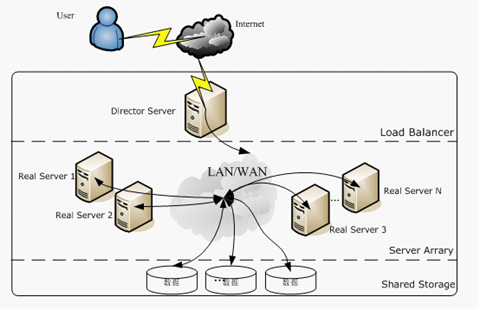潜在语义分析LSA初探

什么是潜在语义分析LSA？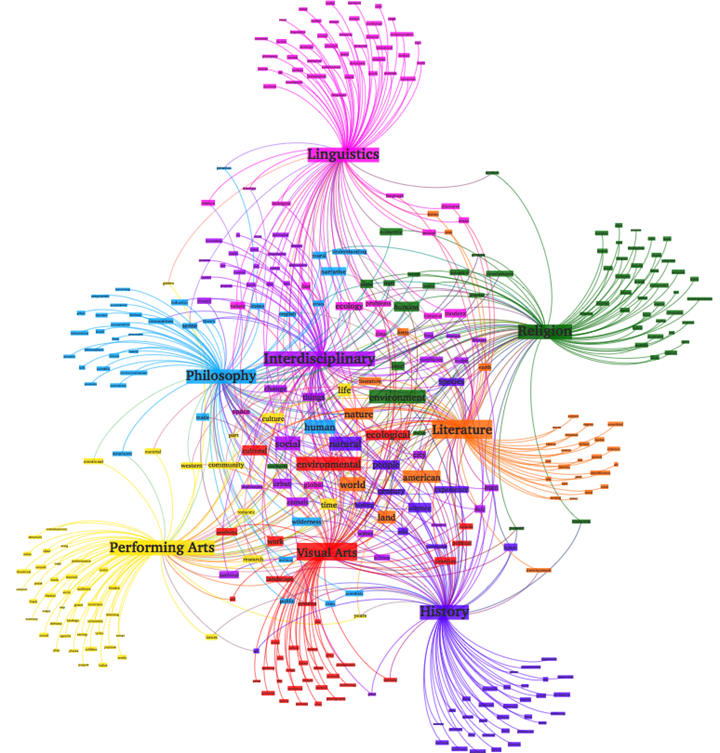• 多义性指的是一个单词可能有多个意思，比如Apple，既可以指水果苹果，也可以指苹果公司
• 同义性指的是多个不同的词可能表示同样的意思，比如search和find。

LSA和传统向量空间模型(vector space model)一样使用向量来表示词(terms)和文档(documents)，并通过向量间的关系(如夹角)来判断词及文档间的关系；不同的是，LSA 将词和文档映射到潜在语义空间，从而去除了原始向量空间中的一些“噪音”，提高了信息检索的精确度。如果两个单词之间有很强的相关性，那么当一个单词出现时，往往意味着另一个单词也应该出现(同义词)；反之，如果查询语句或者文档中的某个单词和其他单词的相关性都不大，那么这个词很可能表示的是另外一个意思(比如在讨论互联网的文章中，Apple更可能指的是Apple公司，而不是水果)。

LSA工具：

• 2009年：Gensim
• 2015年：fastText
• 2016年：text2Vec

潜在语义分析LSA原理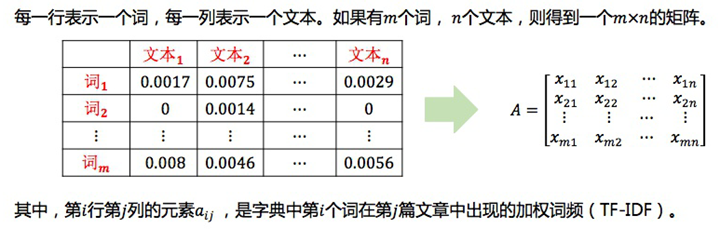LSA试图将原始矩阵降维到一个潜在的概念空间（维度不超过n），然后每个单词或文档都可以用该空间下的一组权值向量（也可认为是坐标）来表示，这些权值反应了与对应的潜在概念的关联程度的强弱。这个降维是通过对该矩阵进行奇异值分解(SVD, singular value decomposition)做到的，计算其用三个矩阵的乘积表示的等价形式，如下：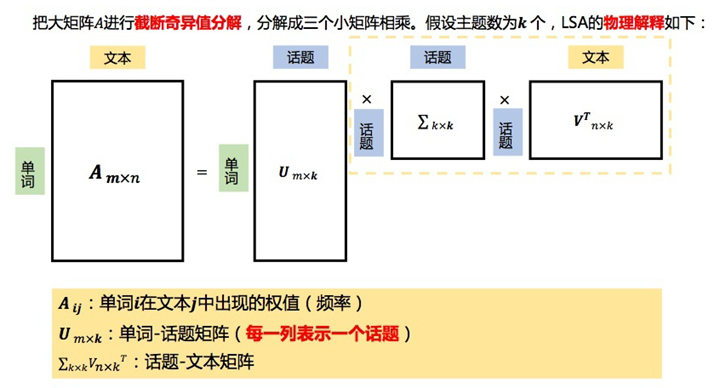LSA的数学原理是矩阵分解（奇异值分解SVD），本质是线性变换（把文本从单词向量空间映射到语义向量空间，词->语义）

潜在语义分析LSA优缺点

LSA的优点：

• 低维空间表示可以刻画同义词，同义词会对应着相同或相似的主题。
• 降维可去除部分噪声，是特征更鲁棒。
• 充分利用冗余数据。
• 无监督/完全自动化。
• 与语言无关。

LSA的缺点：

• LSA可以处理向量空间模型无法解决的一义多词(synonymy)问题，但不能解决一词多义(polysemy)问题。因为LSA将每一个词映射为潜在语义空间中的一个点，也就是说一个词的多个意思在空间中对于的是同一个点，并没有被区分。
• SVD的优化目标基于L-2 norm 或者 Frobenius Norm 的，这相当于隐含了对数据的高斯分布假设。而 term 出现的次数是非负的，这明显不符合 Gaussian 假设，而更接近 Multi-nomial 分布。
• 特征向量的方向没有对应的物理解释。
• SVD的计算复杂度很高，而且当有新的文档来到时，若要更新模型需重新训练。
• 没有刻画term出现次数的概率模型。
• 对于count vectors 而言，欧式距离表达是不合适的（重建时会产生负数）。
• LSA具有词袋模型的缺点，即在一篇文章，或者一个句子中忽略词语的先后顺序。
• LSA的概率模型假设文档和词的分布是服从联合正态分布的，但从观测数据来看是服从泊松分布的。因此LSA算法的一个改进PLSA使用了多项分布，其效果要好于LSA。

潜在语义分析LSA代码示例

# -*- coding: utf-8 -*-
from numpy import zeros
from scipy.linalg import svd
from math import log
import numpy as np
import seaborn as sns
import matplotlib.pyplot as plt
import pandas as pd

class LSA(object):
"""
定义LSA类，w_dict字典用来记录词的个数，d_count用来记录文档号。
"""
def __init__(self, stop_words, ignore_chars):
self.stop_words = stop_words
self.ignore_chars = ignore_chars
self.w_dict = {}
self.d_count = 0

def parse(self, doc):
"""
把文档分词，并滤除停用词和标点，剩下的词会把其出现的文档号填入到w_dict中去，
例如，词book出现在标题3和4中，则我们有self.w_dict[‘book’] = [3, 4]。相当于建了一下倒排。
"""
words = doc.split()
for w in words:
w = w.lower().translate(self.ignore_chars)
if w in self.stop_words:
pass
elif w in self.w_dict:
self.w_dict[w].append(self.d_count)
else:
self.w_dict[w] = [self.d_count]
self.d_count += 1

def build(self):
"""
建立索引词文档矩阵
所有的文档被解析之后，所有出现的词（也就是词典的keys）被取出并且排序。建立一个矩阵，其行数是词的个数，列数是文档个数。
最后，所有的词和文档对所对应的矩阵单元的值被统计出来。
"""
self.keys = [k for k in self.w_dict.keys() if len(self.w_dict[k]) > 1]
self.keys.sort()
self.A = zeros([len(self.keys), self.d_count])
for i, k in enumerate(self.keys):
for d in self.w_dict[k]:
self.A[i, d] += 1

def print_A(self):
"""
打印出索引词文档矩阵。
"""
print(self.A)

def TF_IDF(self):
"""
用TF-IDF替代简单计数
在复杂的LSA系统中，为了重要的词占据更重的权重，原始矩阵中的计数往往会被修改。
最常用的权重计算方法就是TF-IDF（词频-逆文档频率）。基于这种方法，我们把每个单元的数值进行修改。
wordsPerDoc 就是矩阵每列的和,也就是每篇文档的词语总数。DocsPerWord 利用asarray方法创建一个0、1数组（也就是大于0的数值会被归一到1），然后每一行会被加起来，从而计算出每个词出现在了多少文档中。最后，我们对每一个矩阵单元计算TFIDF公式
"""
words_per_doc = np.sum(self.A, axis=0)
docs_per_word = np.sum(np.asarray(self.A > 0, 'i'), axis=1)
rows, cols = self.A.shape
for i in range(rows):
for j in range(cols):
self.A[i, j] = (self.A[i, j] / words_per_doc[j]) * log(float(cols) / docs_per_word[i])

def calc_SVD(self):
"""
建立完词文档矩阵以后，用奇异值分解（SVD）分析这个矩阵。
SVD非常有用的原因是，它能够找到我们矩阵的一个降维表示，他强化了其中较强的关系并且扔掉了噪音（这个算法也常被用来做图像压缩）。
换句话说，它可以用尽可能少的信息尽量完善的去重建整个矩阵。为了做到这点，它会扔掉无用的噪音，强化本身较强的模式和趋势。
利用SVD的技巧就是去找到用多少维度（概念）去估计这个矩阵。太少的维度会导致重要的模式被扔掉，反之维度太多会引入一些噪音。
代码中降到了3维
"""
self.U, self.S, self.Vt = svd(self.A)
target_dimension = 3
self.U2 = self.U[0:, 0:target_dimension]
self.S2 = np.diag(self.S[0:target_dimension])
self.Vt2 = self.Vt[0:target_dimension, 0:]
print("U:\n", self.U2)
print("S:\n", self.S2)
print("Vt:\n", self.Vt2)

def plot_singular_values_bar(self):
"""
为了去选择一个合适的维度数量，我们可以做一个奇异值平方的直方图。它描绘了每个奇异值对于估算矩阵的重要度。
下图是我们这个例子的直方图。（每个奇异值的平方代表了重要程度，下图应该是归一化后的结果）
"""
y_value = (self.S * self.S) / sum(self.S * self.S)
x_value = range(len(y_value))
plt.bar(x_value, y_value, alpha=1, color='g', align="center")
plt.autoscale()
plt.xlabel("Singular Values")
plt.ylabel("Importance")
plt.title("The importance of Each Singular Value")
plt.show()

def plot_singular_heatmap(self):
"""
用颜色聚类
我们可以把数字转换为颜色。例如，下图表示了文档矩阵3个维度的颜色分布。除了蓝色表示负值，红色表示正值，它包含了和矩阵同样的信息。
"""
labels = ["T1", "T2", "T3", "T4", "T5", "T6", "T7", "T8", "T9"]
rows = ["Dim1", "Dim2", "Dim3"]
self.Vt_df_norm = pd.DataFrame(self.Vt2 * (-1))
self.Vt_df_norm.columns = labels
self.Vt_df_norm.index = rows
sns.set(font_scale=1.2)
ax = sns.heatmap(self.Vt_df_norm, cmap=plt.cm.bwr, linewidths=.1, square=2)
ax.xaxis.tick_top()
plt.xlabel("Book Title")
plt.ylabel("Dimensions")
plt.show()

if __name__ == '__main__':
# 待处理的文档
titles = [
"The Neatest Little Guide to Stock Market Investing",
"Investing For Dummies, 4th Edition",
"The Little Book of Common Sense Investing: The Only Way to Guarantee Your Fair Share of Stock Market Returns",
"The Little Book of Value Investing",
"Value Investing: From Graham to Buffett and Beyond",
"Rich Dad's Guide to Investing: What the Rich Invest in, That the Poor and the Middle Class Do Not!",
"Investing in Real Estate, 5th Edition",
"Stock Investing For Dummies",
"Rich Dad's Advisors: The ABC's of Real Estate Investing: The Secrets of Finding Hidden Profits Most Investors Miss"
]
# 定义停止词
stopwords = ['and', 'edition', 'for', 'in', 'little', 'of', 'the', 'to']
# 定义要去除的标点符号
ignore_chars = ''',:'!'''

mylsa = LSA(stopwords, ignore_chars)
for t in titles:
mylsa.parse(t)
mylsa.build()
mylsa.print_A()
mylsa.TF_IDF()
mylsa.print_A()
mylsa.calc_SVD()
mylsa.plot_singular_values_bar()
mylsa.plot_singular_heatmap()

import pandas as pd
import matplotlib.pyplot as plt
from nltk.corpus import stopwords
from sklearn.feature_extraction.text import TfidfVectorizer
from sklearn.datasets import fetch_20newsgroups
from sklearn.decomposition import TruncatedSVD
import umap

dataset = fetch_20newsgroups(shuffle=True, random_state=1, remove=('headers', 'footers', 'quotes'))
documents = dataset.data
print(len(documents))
print(dataset.target_names)

news_df = pd.DataFrame({'document': documents})

# remove everything except alphabets`
news_df['clean_doc'] = news_df['document'].replace("[^a-zA-Z]", " ")

# remove short words
news_df['clean_doc'] = news_df['clean_doc'].apply(lambda x: ' '.join([w for w in x.split() if len(w) > 3]))

# make all text lowercase-
news_df['clean_doc'] = news_df['clean_doc'].apply(lambda x: x.lower())

# tokenization
tokenized_doc = news_df['clean_doc'].apply(lambda x: x.split())

# remove stop-words
stop_words = stopwords.words('english')
tokenized_doc = tokenized_doc.apply(lambda x: [item for item in x if item not in stop_words])

# de-tokenization
detokenized_doc = []
for i in range(len(news_df)):
t = ' '.join(tokenized_doc[i])
detokenized_doc.append(t)

news_df['clean_doc'] = detokenized_doc

vectorizer = TfidfVectorizer(stop_words='english', max_features=1000, max_df=0.5, smooth_idf=True)
X = vectorizer.fit_transform(news_df['clean_doc'])
print(X.shape)

# SVD represent documents and terms in vectors
svd_model = TruncatedSVD(n_components=20, algorithm='randomized', n_iter=100, random_state=122)
svd_model.fit(X)
print(len(svd_model.components_))

terms = vectorizer.get_feature_names()
for i, comp in enumerate(svd_model.components_):
terms_comp = zip(terms, comp)
sorted_terms = sorted(terms_comp, key=lambda x: x,reverse=True)[:7]
sorted_terms_words = [t for t in sorted_terms]
print("Topic " + str(i) + ": " + str(sorted_terms_words))

X_topics = svd_model.fit_transform(X)
embedding = umap.UMAP(n_neighbors=150, min_dist=0.5, random_state=12).fit_transform(X_topics)
plt.figure(figsize=(7, 5))
plt.scatter(embedding[:, 0], embedding[:, 1], c=dataset.target, s=10, edgecolor='none')
plt.show()

潜在语义分析实战：基于LSA的情感分类

• Id：自增长ID，无含义
• ProductId：产品ID
• UserId：会员ID
• ProfileName：会员昵称
• Score：点评分
• Time：点评时间
• Summary：综合评价
• Text：点评详情

import numpy as np
import pandas as pd
from sklearn.feature_extraction.text import TfidfVectorizer
from sklearn.model_selection import train_test_split
from sklearn.feature_extraction.text import CountVectorizer
from sklearn.ensemble import RandomForestClassifier
from sklearn.pipeline import Pipeline
from sklearn.metrics import accuracy_score
from sklearn.metrics import classification_report
from sklearn.feature_selection import chi2
import matplotlib.pyplot as plt

df.dropna(inplace=True)
df[df['Score'] != 3]
df['Positivity'] = np.where(df['Score'] > 3, 1, 0)
X = df['Text']
y = df['Positivity']
X_train, X_test, y_train, y_test = train_test_split(X, y, test_size=0.25, random_state=0)
print("Train set has total {0} entries with {1:.2f}% negative, {2:.2f}% positive".format(
len(X_train),
(len(X_train[y_train == 0]) / (len(X_train) * 1.)) * 100,
(len(X_train[y_train == 1]) / (len(X_train) * 1.)) * 100)
)

def accuracy_summary(pipeline, X_train, y_train, X_test, y_test):
sentiment_fit = pipeline.fit(X_train, y_train)
y_pred = sentiment_fit.predict(X_test)
accuracy = accuracy_score(y_test, y_pred)
print("accuracy score: {0:.2f}%".format(accuracy * 100))
return accuracy

cv = CountVectorizer()
rf = RandomForestClassifier(class_weight="balanced")
n_features = np.arange(10000, 30001, 10000)

def nfeature_accuracy_checker(vectorizer=cv, n_features=n_features, stop_words=None, ngram_range=(1, 1), classifier=rf):
result = []
print(classifier)
for n in n_features:
vectorizer.set_params(stop_words=stop_words, max_features=n, ngram_range=ngram_range)
checker_pipeline = Pipeline([
('vectorizer', vectorizer),
('classifier', classifier)
])
print("Test result for {} features".format(n))
nfeature_accuracy = accuracy_summary(checker_pipeline, X_train, y_train, X_test, y_test)
result.append((n, nfeature_accuracy))
return result

tfidf = TfidfVectorizer()
feature_result_tgt = nfeature_accuracy_checker(vectorizer=tfidf, ngram_range=(1, 3))

cv = CountVectorizer(max_features=30000, ngram_range=(1, 3))
pipeline = Pipeline([
('vectorizer', cv),
('classifier', rf)
])
sentiment_fit = pipeline.fit(X_train, y_train)
y_pred = sentiment_fit.predict(X_test)
print(classification_report(y_test, y_pred, target_names=['negative', 'positive']))

tfidf = TfidfVectorizer(max_features=30000, ngram_range=(1, 3))
X_tfidf = tfidf.fit_transform(df.Text)
y = df.Positivity
chi2score = chi2(X_tfidf, y)

plt.figure(figsize=(12, 8))
scores = list(zip(tfidf.get_feature_names(), chi2score))
chi2 = sorted(scores, key=lambda x: x)
topchi2 = list(zip(*chi2[-20:]))
x = range(len(topchi2))
labels = topchi2
plt.barh(x, topchi2, align='center', alpha=0.5)
plt.plot(topchi2, x, '-o', markersize=5, alpha=0.8)
plt.yticks(x, labels)
plt.xlabel('$\chi^2$')
plt.show()

潜在语义分析LSA的进化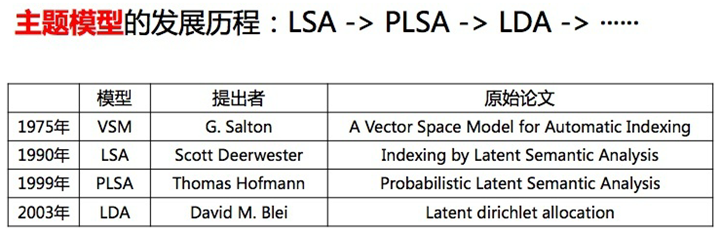LSA

LSA 方法快速且高效，但它也有一些主要缺点：

• 缺乏可解释的嵌入（我们并不知道主题是什么，其成分可能积极或消极，这一点是随机的）
• 需要大量的文件和词汇来获得准确的结果
• 表征效率低

pLSA

pLSA，即概率潜在语义分析，采取概率方法替代 SVD 以解决问题。其核心思想是找到一个潜在主题的概率模型，该模型可以生成我们在文档-术语矩阵中观察到的数据。特别是，我们需要一个模型 P(D,W)，使得对于任何文档 d 和单词 w，P(d,w) 能对应于文档-术语矩阵中的那个条目。

• 给定文档 d，主题 z 以 P(z|d) 的概率出现在该文档中
• 给定主题 z，单词 w 以 P(w|z) 的概率从主题 z 中提取出来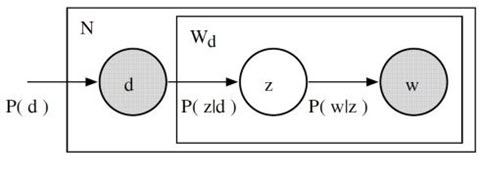$$P(D,W)=P(D)\sum_{Z}P(Z|D)P(W|Z)$$

$$P(D,W)=\sum_{Z}P(Z)P(Z|D)P(W|Z)$$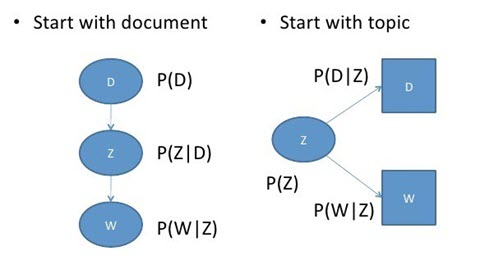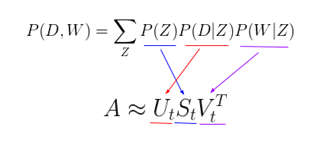• 因为没有参数来给 P(D) 建模，所以不知道如何为新文档分配概率
• pLSA 的参数数量随着我们拥有的文档数线性增长，因此容易出现过度拟合问题

LDA

LDA 即潜在狄利克雷分布，是 pLSA 的贝叶斯版本。它使用狄利克雷先验来处理文档-主题和单词-主题分布，从而有助于更好地泛化。我们可以对狄利克雷分布其做一个简短的概述：即，将狄利克雷视为「分布的分布」。本质上，它回答了这样一个问题：「给定某种分布，我看到的实际概率分布可能是什么样子？」考虑比较主题混合概率分布的相关例子。假设我们正在查看的语料库有着来自 3 个完全不同主题领域的文档。如果我们想对其进行建模，我们想要的分布类型将有着这样的特征：它在其中一个主题上有着极高的权重，而在其他的主题上权重不大。如果我们有 3 个主题，那么我们看到的一些具体概率分布可能会是：

• 混合 X：90% 主题 A，5% 主题 B，5% 主题 C
• 混合 Y：5% 主题 A，90% 主题 B，5% 主题 C
• 混合 Z：5% 主题 A，5% 主题 B，90% 主题 C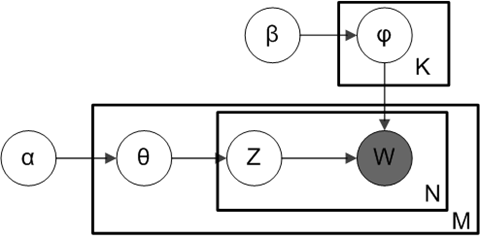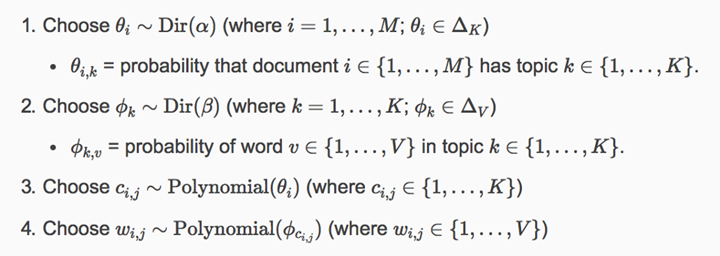LDA 无疑是最受欢迎（且通常来说是最有效的）主题建模技术。它在 gensim 当中可以方便地使用：

from gensim.corpora import MmCorpus
from gensim.models.ldamodel import LdaModel

document = "This is some document..."

# load id->word mapping (the dictionary)

mm = MmCorpus('wiki_en_tfidf.mm')

# extract 100 LDA topics, updating once every 10,000
lda = LdaModel(corpus=mm, id2word=id2word, num_topics=100, update_every=1, chunksize=10000, passes=1)

# use LDA model: transform new doc to bag-of-words, then apply lda
doc_bow = doc2bow(document.split())
doc_lda = lda[doc_bow]

# doc_lda is vector of length num_topics representing weighted presence of each topic in the doc

深度学习中的 LDA：lda2vec

lda2vec 专门在 word2vec 的 skip-gram 模型基础上建模，以生成单词向量。skip-gram 和 word2vec 本质上就是一个神经网络，通过利用输入单词预测周围上下文词语的方法来学习词嵌入。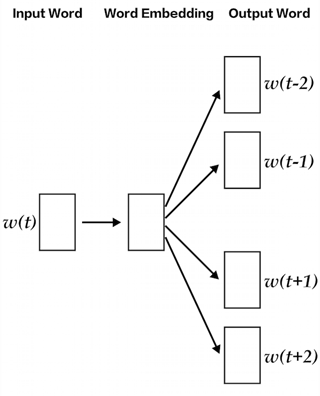• 文档权重向量，表示文档中每个主题的「权重」（稍后将转换为百分比）
• 主题矩阵，表示每个主题及其相应向量嵌入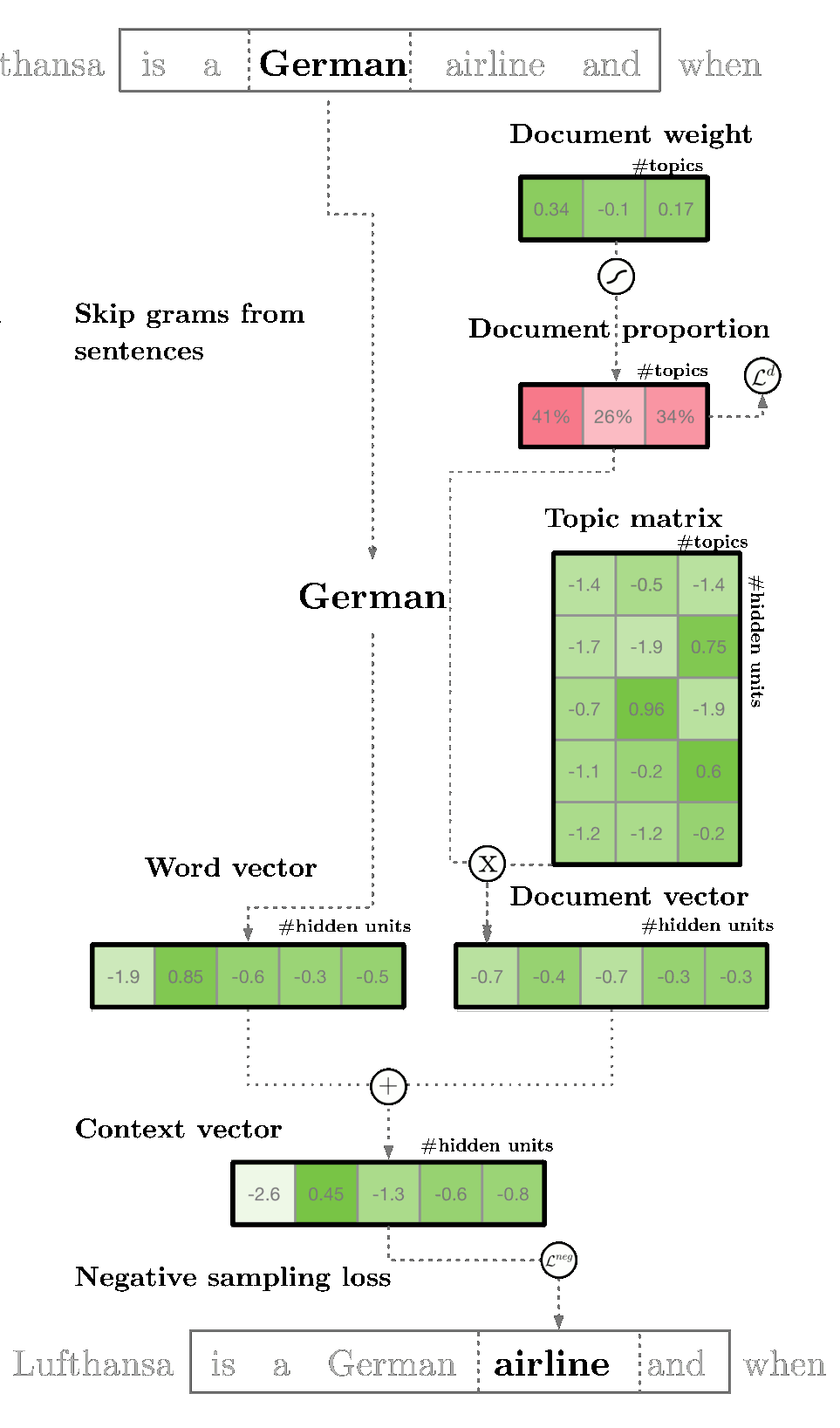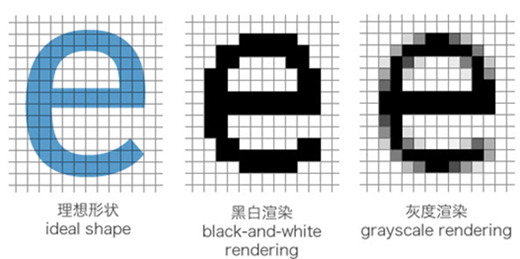网站与APP开发中的字体设置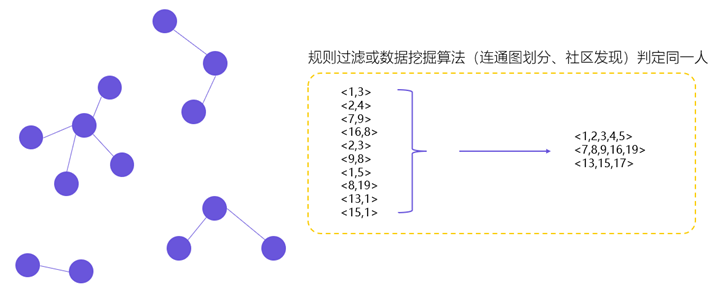用户体系搭建之ID-Mapping